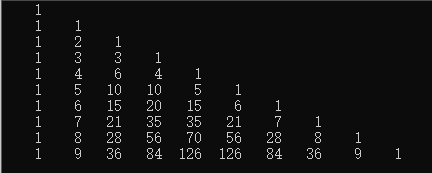• 本文实例讲述了C语言实现杨辉三角的方法，分享给大家供大家参考。 具体实现方法如下： #include using namespace std; void printYangHui(int line) { int **array = new int*[line]; for (int i = 0; i < ...
• ## 杨辉三角形c语言程序

万次阅读 多人点赞 2020-08-03 11:53:19
杨辉三角形 #include <stdio.h> #define N 7 int main() { int i,j; int a[N][N]; for(i = 0; i < N; i++) //杨辉三角形的每一行的首位都是1 { a[i][i] = 1; a[i] = 1; } for(i = 2; i < ...
杨辉三角形
#include <stdio.h>
#define N 7

int main()
{
int i,j;
int a[N][N];

for(i = 0; i < N; i++) //杨辉三角形的每一行的首位都是1
{
a[i][i] = 1;
a[i] = 1;
}

for(i = 2; i < N; i++)
{
for(j = 1; j <= i - 1; j++)
{
a[i][j] = a[i-1][j-1] + a[i-1][j];
}
}

for(i = 0; i < N; i++)
{
for(j = 0; j <=i; j++)
{
printf("%6d", a[i][j]);

}
printf("\n");
}
printf("\n");
return 0;
}


展开全文• 杨辉三角是我们初学C语言就知道的，现在，让我们用C语言将它在计算机上显示出来。 在初中，我们就知道，杨辉三角的两个腰边的数都是1，其它位置的数都是上顶上两个数之和。这就是我们用C语言杨辉三角的关键之一。...
第一版：一个二维数组
第二版：两个一维数组
第三版：一个一维数组
时间复杂度都为
但空间复杂度分别为,,,依次减少 我们知道，杨辉三角的两个腰边的数都是1，其它位置的数都是上顶上两个数之和。这就是我们用C语言写杨辉三角的关键之一。这句是关键arr[i][j]=arr[i-1][j]+arr[i-1][j-1];
最简单的代码如下
#include<stdio.h>
const int n=10;
void Yang_Hui_Triangle(int (*arr),int col,int row)
{
for(int i=0;i<col;i++)
{
for(int j=0;j<=i;j++)
{
if(i==j||j==0)
{
arr[i][j]=1;
}
else
{
arr[i][j]=arr[i-1][j]+arr[i-1][j-1];
}
}
}
}

void Print_YHC(int (*arr),int col,int row)
{
for(int i=0;i<col;i++)
{
for(int j=0;j<=i;j++)
{
printf("%5d",arr[i][j]);
}
printf("\n");
}
}
int main()
{

int arr[n][n]={0};
Yang_Hui_Triangle(arr,n,n);
Print_YHC(arr,n,n);
return 0;
}结果是正确的，但有一半的空间被浪费了。
但假如我们不知到，需要打印的结束那怎么办呢？有人会说，我们可以现要求输入，在创建数组呀
	int n=0;
scanf("%d",&n);
int arr[n][n]={0};
理论上这也不是不可以，但可惜有的编译器不支持啊。
我们可以在详细看一下题目，题目要求的是打印，而不需要存储，而杨辉三角的一行仅与上一行有关，我们就可以想到用两个一维数组，交替进行计算并，输出与存储，来打印N阶的杨辉三角。代码如下
#include<stdio.h>
#include<stdlib.h>
void Yang_Hui_Triangle(int order)
{
int *p=(int*)malloc(sizeof(int)*(order+1));
int *q=(int*)malloc(sizeof(int)*(order+1));
//应检查p,q是否申请成功
for (int i = 0;i < order;i++)
{
for (int j = 0;j <= i;j++)
{
if (i == j || j == 0)
{
*(q + j) = 1;
printf("%5d", *(q + j));
}
else
{
*(q + j) = *(p + j) + *(p + j - 1);
printf("%5d", *(q + j));
}
}
printf("\n");
p = q;
}
}

int main()
{
int order = 0;
scanf_s("%d", &order);
Yang_Hui_Triangle(order);
return 0;
}
这样我们节省了大部分不需要的空间。
当然还能再省，只用一个数组即可，通过观察，每个普遍位置arr[n][i]=arr[n-1][i]+arr[n-1][i-1],其他位置都是1.
。
#include<stdio.h>
#include<stdlib.h>
void Yang_Hui_Triangle(int order)
{
int* p = (int*)malloc(sizeof(int) * (order + 1));
//应检查p，是否申请成功
for (int i = 0; i < order; i++)
{
for (int j = i; j >= 0; j--)
{
if (i == j || j == 0)
{
*(p + j) = 1;
printf("%5d", *(p + j));
}
else
{
*(p + j) = *( p + j - 1) + *(p + j);
printf("%5d", *(p + j));
}
}
printf("\n");
}
}

int main()
{
int order = 0;
scanf_s("%d", &order);
Yang_Hui_Triangle(order);
return 0;
}

展开全文程序设计
• 1130: 杨辉三角 时间限制: 1 Sec 内存限制: 128 MB 提交: 11708 解决: 6696 [状态] [讨论版] [提交] [命题人:admin] 题目描述 还记得中学时候学过的杨辉三角吗？具体的定义这里不再描述，你可以参考以下的图形： 1 1...
1130: 杨辉三角 时间限制: 1 Sec 内存限制: 128 MB 提交: 11708 解决: 6696 [状态] [讨论版] [提交] [命题人:admin] 题目描述 还记得中学时候学过的杨辉三角吗？具体的定义这里不再描述，你可以参考以下的图形： 1 1 1 1 2 1 1 3 3 1 1 4 6 4 1 1 5 10 10 5 1
输入 输入只包含一个正整数n（1 <= n <= 30），表示将要输出的杨辉三角的层数。
输出 输出相应层数的杨辉三角，每一层的整数之间用一个空格隔开。
样例输入 Copy 4 样例输出 Copy 1 1 1 1 2 1 1 3 3 1
#include <stdio.h>
#include <stdlib.h>

#define N 31

//杨辉三角
int main() {
int i, j, n;
int a[N][N];

scanf("%d", &n);
a = a = a = 1;

//0列和对角线元素都为1
for(i = 2; i < n; i++)
a[i] = a[i][i] = 1;

//计算其余位的元素
for(i = 2; i < n; i++){
for(j = 1; j < i; j++){
a[i][j] = a[i - 1][j] + a[i - 1][j - 1];
}
}

//输出杨辉三角
for(i = 0; i < n; i++){
for(j = 0; j <= i; j++){
printf("%d ", a[i][j]);
}
printf("\n");
}
return 0;
}

展开全文• printf("请输入杨辉三角规模:\n"); scanf("%d",&N); EnQueue(Q,1); for(n=2;n;n++){ EnQueue(Q,1); for(int c=1;c;c++){ t=GetHead(Q); DeQueue(Q); printf("%4d",t); x=GetHead(Q); t=t+x; EnQueue(Q,t); } ...
#include<stdio.h>
#include<stdlib.h>

#define MAXQSIZE 200

typedef int QElemType;

typedef struct {
QElemType  *base;
int front;
int rear;
}SqQueue;

//构造一个空队列Q
void InitQueue(SqQueue *Q)
{
Q->base=(QElemType *)malloc(MAXQSIZE*sizeof(QElemType));
if(!Q->base)
exit(1);
Q->front=Q->rear=0;
}

//返回Q的元素个数，即队列的长度
int QueueLength(SqQueue *Q)
{
int e;
e=(Q->rear-Q->front+MAXQSIZE)%MAXQSIZE;
return e;
}

//插入的元素e为Q的新的队尾元素
void EnQueue(SqQueue *Q,QElemType e)
{
if((Q->rear+1)%MAXQSIZE ==Q->front)
exit(1);
Q->base[Q->rear]=e;
Q->rear=(Q->rear+1)%MAXQSIZE;
}

//若队列不空，则删除Q的队头元素，用e返回其值
void DeQueue(SqQueue *Q)
{
if(Q->front==Q->rear)
exit(1);
//e=Q.base[Q.front];
Q->front=(Q->front+1)%MAXQSIZE;
}

//返回队头元素
{
return Q->base[Q->front];
}

//主函数
int main()
{
int N,n;
QElemType t,x;
SqQueue f,*Q;
Q=&f;
InitQueue(Q);
printf("请输入杨辉三角规模:\n");
scanf("%d",&N);
EnQueue(Q,1);
for(n=2;n<=N;n++){
EnQueue(Q,1);
for(int c=1;c<=n-2;c++){
DeQueue(Q);
printf("%4d",t);
t=t+x;
EnQueue(Q,t);
}
EnQueue(Q,1);
DeQueue(Q);
printf("\n");
}
while(Q->front!=Q->rear){
DeQueue(Q);
}
printf("\n");
return 0;
}


展开全文队列 数据结构
• 杨辉三角形又称Pascal三角形，它的第i+1行是(a+b)i的展开式的系数。 它的一个重要性质是：三角形中的每个数字等于它两肩上的数字相加。 下面给出了杨辉三角形的前4行： 1 1 1 1 2 1 1 3 3 1 给出n，输出它的前n行。 ...算法
• 杨辉三角 C语言【一维数组】 说明 写《C语言程序设计》作业的时候想到的一个算法，只用一维数组就可以实现杨辉三角的输出（不过肯定没用存储的功能了），自己感觉挺妙的，在答案和其他地方也没看到一样的，传上来...
• 杨辉三角 C语言 问题描述 杨辉三角形又称Pascal三角形，它的第i+1行是(a+b)i的展开式的系数。 它的一个重要性质是：三角形中的每个数字等于它两肩上的数字相加。 下面给出了杨辉三角形的前4行： 1 1 1 1 2 1 ...蓝桥杯
• 打印杨辉三角 输入描述： 第一行包含一个整数数n。 (1≤n≤30) 输出描述： 包含n行，为杨辉三角的前n行。 例如： 输入：6 输出： 1 1 1 1 2 1 1 3 3 1 = 1 4 6 4 1 1 5 10 10 5 1 思路： 很多同学...
• ## 基础练习 杨辉三角形 c语言

千次阅读 多人点赞 2019-06-29 17:10:18
基础练习 杨辉三角形 问题描述 杨辉三角形又称Pascal三角形，它的第i+1行是(a+b)i的展开式的系数。 它的一个重要性质是：三角形中的每个数字等于它两肩上的数字相加。 下面给出了杨辉三角形的前4行： 1 1 1 1 2 1 1 ...c
• ## 杨辉三角C语言编程

万次阅读 多人点赞 2018-07-17 22:36:04
杨辉三角在程序练习当中是比较常见的，根据上面的给出的数列可以看出一些规律来，每行的两边都是1，从第2列开始，每个数是上一行相邻的数之和，根据自己的经验编写一个输出10行的C语言函数，如下： #include &...
• ## 杨辉三角C语言 SDUT

千次阅读 2019-01-07 19:27:30
杨辉三角 Time Limit: 1000 ms Memory Limit: 65536 KiB Submit Statistic Problem Description 1  1 1 1 2 1 1 3 3 1 1 4 6 4 1 1 5 10 10 5 1  上面的图形熟悉吗？它就是我们中学时候学过的杨辉...
• #include<stdio.h> int main(){ int i,j; int a; int n; scanf("%d",&n); for(i=0;i<n;i++){ for(j=0;j<=i;j++){ if(i==j||j==0) a[i][j]=1; else...
• C语言编写在控制台上输出杨辉三角。用的是txt格式保存c 算法
• 杨辉三角 问题描述 用函数编程计算并输出如图所示的杨辉三角形。 1 1 1 1 2 1 1 3 3 1 1 4 6 4 1 1 5 10 10 5 1 1 6 15 20 15 6 1 解答 #include <stdio.h> #include <stdlib.h> int main() { int a...
• 很小的一个程序，也很简练，易懂，打印出杨辉三角形
• 想必大家在初高中的时候一定都学过杨辉三角吧，这是一个对几何学和代数学都十分重要的东西，他的行列之间都有一定的规律，现在用c语言杨辉三角实现，具体代码如下： 我们都知道杨辉三角的特性，它的第一列和...html
• void main() { int i; int j; int arr; for(i=0; i<10; i++) { arr[i] = 1; arr[i][i] = 1; } for(i=2; i<10;i++) { for(j=1; j<=i-1;j++) { arr[i][j] = arr[i-1][j] + arr[i-1][j-1];...for...
• title: C语言实现杨辉三角 date: 2020-04-29 tags: C语言 categories: 编程 杨辉三角的特点，每一行的第一列和最后一列都是1，并且上面一行的俩个数相加等于下面一行的一个数 代码如下： #include<stdio.h> ...算法
• 题目 leetcode 118 代码 int** generate(int numRows, int* returnSize, int** returnColumnSizes){ *returnSize = 0; int **res = (int **)malloc(sizeof(int *) * numRows); (*returnColumnSizes) = (in...
• #include<stdio.h> int main() { int n,a; scanf("%d",&n); int i,j; for(i=0;i<n+1;i++)//定义三角形两边都为1 { a[i]=1; a[i][i]=1; } for(i=2;... for(j...
• 输出10行杨辉三角——C语言C语言】 代码如下： #include<stdio.h> //预处理指令 #include<stdlib.h> //标准库头文件 void main() //定义函数类型 { int a; //定义一个整形型二维数组 ...数据结构...

# 杨辉三角c语言c语言 订阅